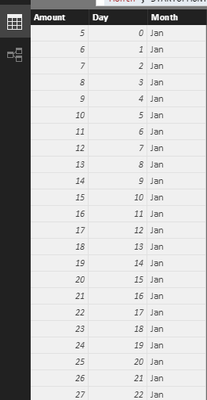cancel
Showing results for
Did you mean:Frequent Visitor

## Weeks after the beginning of month

Hi guys,

I would like to compare quality of customers by month. On Y-axis should be values, on X-axis - days (weeks) after the beginning of a month (from 1 up till 365 or from 1 up till 52), in legend - months. The idea is simply to look at the quality of customers in the beginning of each month and then moving forward. I'm really struggling to figure our how to make this "days/weeks after beginning of a month" thing happen and looking forward for your help.

Attaching a graph screenshot made in excel for your better understanding what i'm trying to do1 ACCEPTED SOLUTIONMicrosoft

Actually, your requirement cannot be achieved in a line chart. Because you were attempting to display some data that is not existing in a month. For example, for February it will show up to 48 days starting from 0, while in February there are only 28 data rows, the data from day 29 to day 48 comes from March, however, in a chart, under each group instance (Month), it can not show the non-existing data values.

If you indeed want that output, you can try below workaround. It would be complex if there are many data records in table view.

The sample table used in my test contains two columns, [Date] and [Amount].

I added some calculated columns as below:

```Day for Jan =
DATEDIFF ( MIN ( 'STARTOFMONTH'[Date] ), MAX ( 'STARTOFMONTH'[Date] ), DAY )
- DATEDIFF ( 'STARTOFMONTH'[Date], MAX ( 'STARTOFMONTH'[Date] ), DAY )

Jan = "Jan"

Day for Feb =
IF (
'STARTOFMONTH'[Date].[MonthNo] >= 2,
DATEDIFF (
MINX (
FILTER ( 'STARTOFMONTH', 'STARTOFMONTH'[Date].[MonthNo] = 2 ),
'STARTOFMONTH'[Date]
),
MAX ( 'STARTOFMONTH'[Date] ),
DAY
)
- DATEDIFF ( 'STARTOFMONTH'[Date], MAX ( 'STARTOFMONTH'[Date] ), DAY ),
BLANK ()
)

Feb = "Feb"```Then, create a calculated table.

```Table =
UNION (
SELECTCOLUMNS (
'STARTOFMONTH',
"Amount", 'STARTOFMONTH'[Amount],
"Day", 'STARTOFMONTH'[Day for Jan],
"Month", 'STARTOFMONTH'[Jan]
),
SELECTCOLUMNS (
'STARTOFMONTH',
"Amount", 'STARTOFMONTH'[Amount],
"Day", 'STARTOFMONTH'[Day for Feb],
"Month", 'STARTOFMONTH'[Feb]
)
)
```In a line chart visual, add Table[Amount] as Values, add Table[Day] as Axis, add Table[Month] as Legend.Best regards,
Yuliana Gu

Community Support Team _ Yuliana Gu
If this post helps, then please consider Accept it as the solution to help the other members find it more quickly.
4 REPLIES 4Microsoft

Actually, your requirement cannot be achieved in a line chart. Because you were attempting to display some data that is not existing in a month. For example, for February it will show up to 48 days starting from 0, while in February there are only 28 data rows, the data from day 29 to day 48 comes from March, however, in a chart, under each group instance (Month), it can not show the non-existing data values.

If you indeed want that output, you can try below workaround. It would be complex if there are many data records in table view.

The sample table used in my test contains two columns, [Date] and [Amount].

I added some calculated columns as below:

```Day for Jan =
DATEDIFF ( MIN ( 'STARTOFMONTH'[Date] ), MAX ( 'STARTOFMONTH'[Date] ), DAY )
- DATEDIFF ( 'STARTOFMONTH'[Date], MAX ( 'STARTOFMONTH'[Date] ), DAY )

Jan = "Jan"

Day for Feb =
IF (
'STARTOFMONTH'[Date].[MonthNo] >= 2,
DATEDIFF (
MINX (
FILTER ( 'STARTOFMONTH', 'STARTOFMONTH'[Date].[MonthNo] = 2 ),
'STARTOFMONTH'[Date]
),
MAX ( 'STARTOFMONTH'[Date] ),
DAY
)
- DATEDIFF ( 'STARTOFMONTH'[Date], MAX ( 'STARTOFMONTH'[Date] ), DAY ),
BLANK ()
)

Feb = "Feb"```Then, create a calculated table.

```Table =
UNION (
SELECTCOLUMNS (
'STARTOFMONTH',
"Amount", 'STARTOFMONTH'[Amount],
"Day", 'STARTOFMONTH'[Day for Jan],
"Month", 'STARTOFMONTH'[Jan]
),
SELECTCOLUMNS (
'STARTOFMONTH',
"Amount", 'STARTOFMONTH'[Amount],
"Day", 'STARTOFMONTH'[Day for Feb],
"Month", 'STARTOFMONTH'[Feb]
)
)
```In a line chart visual, add Table[Amount] as Values, add Table[Day] as Axis, add Table[Month] as Legend.Best regards,
Yuliana Gu

Community Support Team _ Yuliana Gu
If this post helps, then please consider Accept it as the solution to help the other members find it more quickly.Frequent Visitor

Brilliant, thanks!Helper I

DAX to the rescue! 🙂 Just create a calculated column in your date table like this:

days after start of month = DATEDIFF(STARTOFMONTH(Dates[Date]), Dates[Date], DAY)

That should do the trick.Frequent Visitor

Hi chbraun, thanks a lot for your response!

Your solution works for comparison, but it will show only the days from 1 to 30 for each month.

Is there any way to present graphically the information with ALL the days after the beginning of each month? For instance, now is 20.03.2017 so for March the line graph will show the trend for 20 days starting from 0, for February it will show up to 48 days starting from 0, etc. Attaching the screenshot of what I have in mind:)